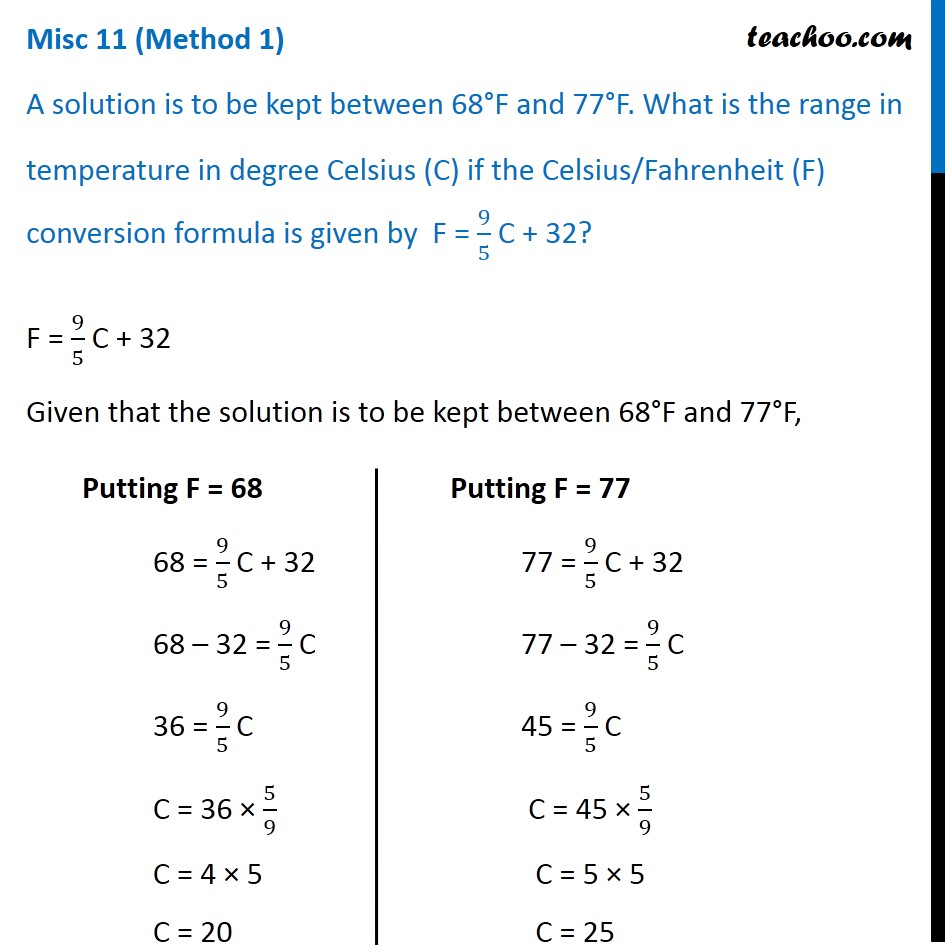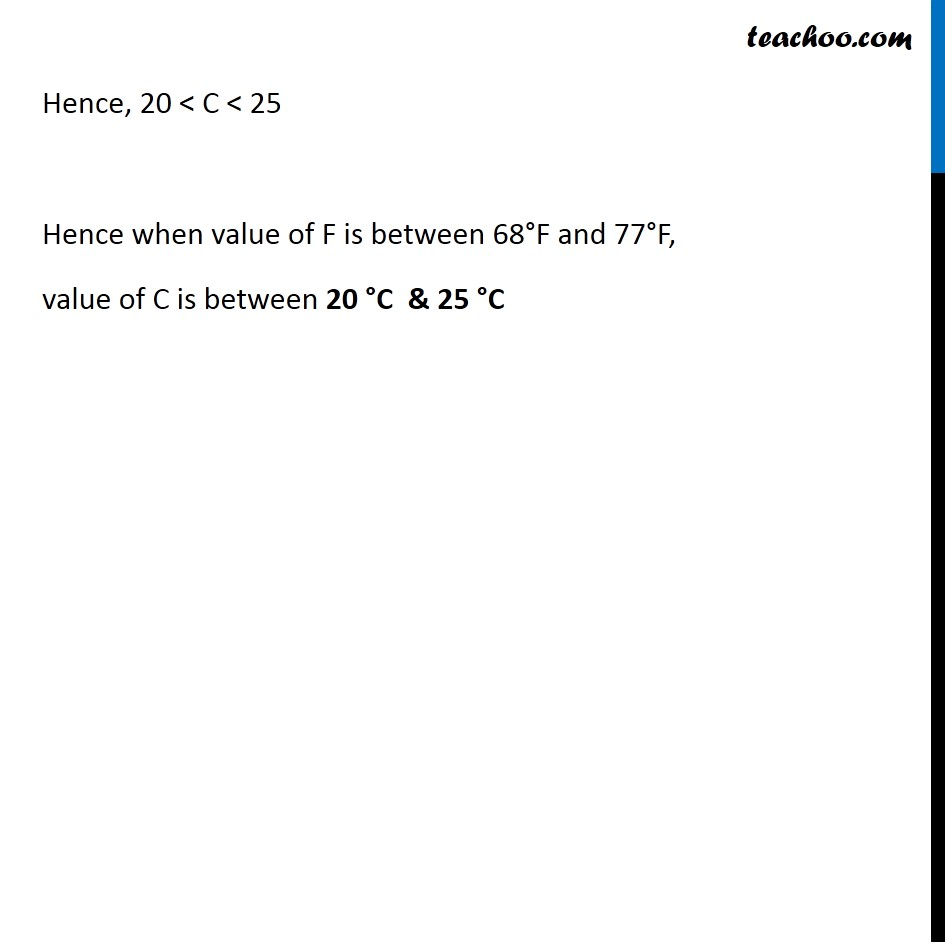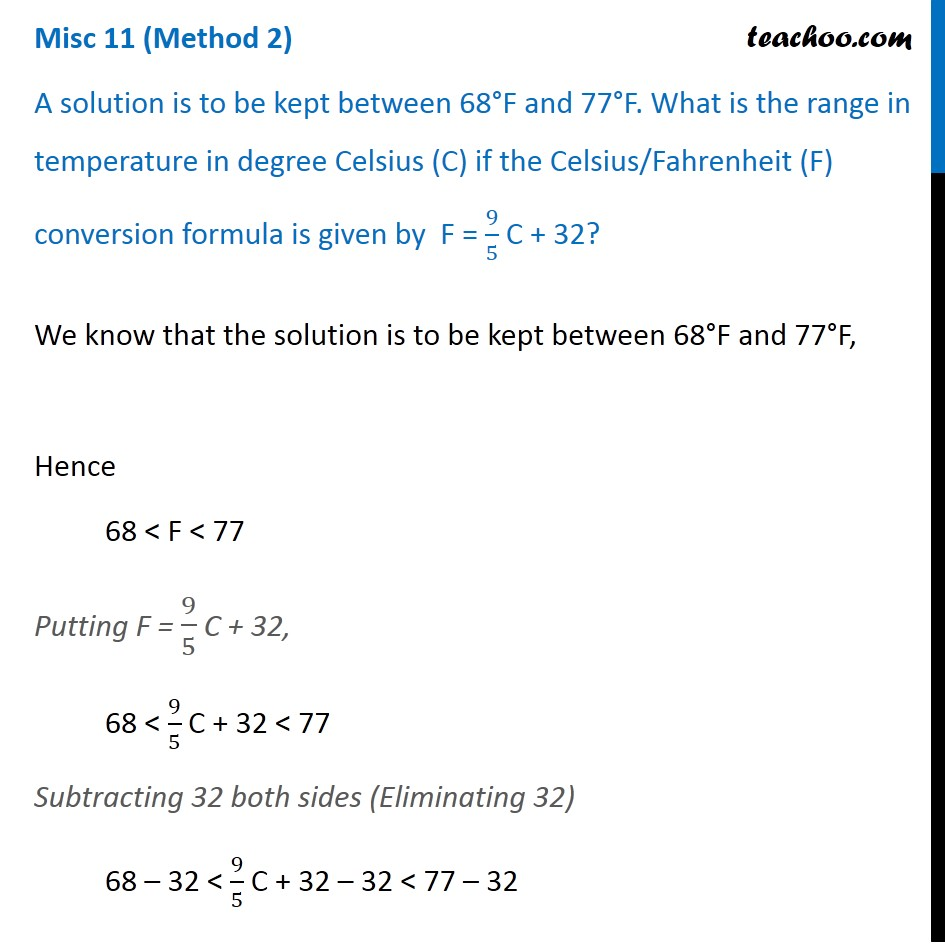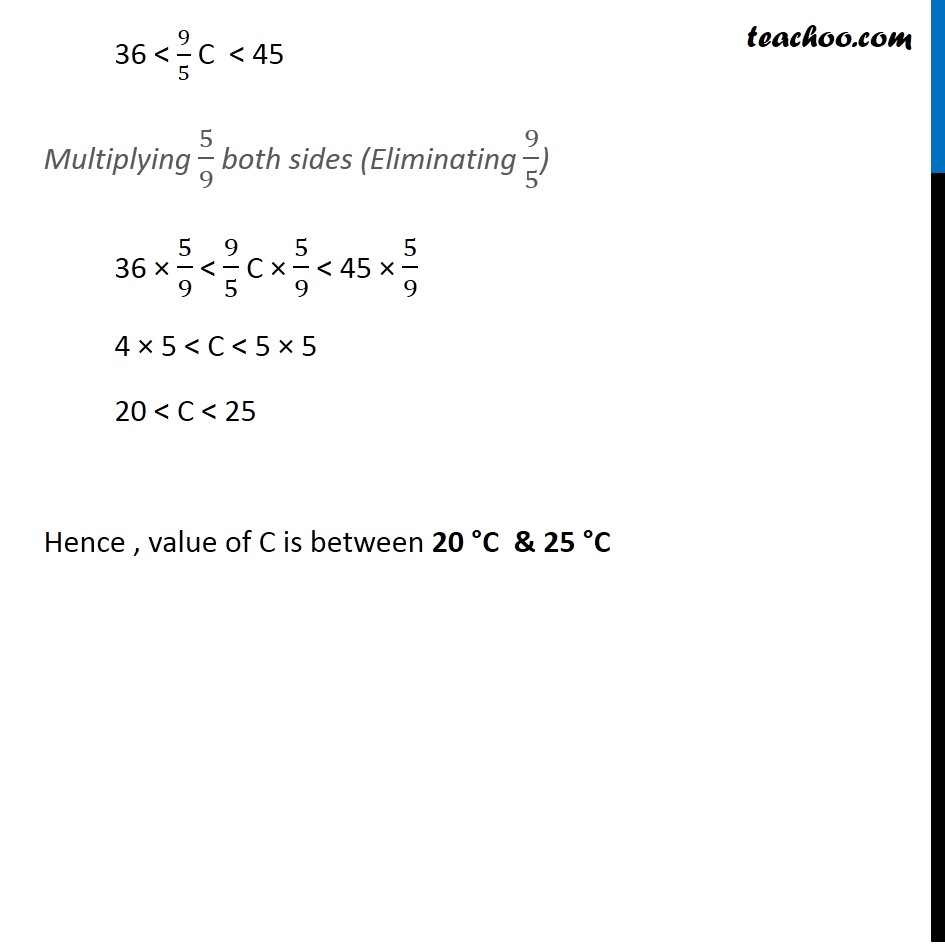Miscellaneous

Chapter 5 Class 11 Linear Inequalities
Serial order wiseLearn in your speed, with individual attention - Teachoo Maths 1-on-1 Class

### Transcript

Misc 11 (Method 1) A solution is to be kept between 68°F and 77°F. What is the range in temperature in degree Celsius (C) if the Celsius/Fahrenheit (F) conversion formula is given by F = 9/5 C + 32? F = 9/5 C + 32 Given that the solution is to be kept between 68°F and 77°F, Putting F = 68 68 = 9/5 C + 32 68 – 32 = 9/5 C 36 = 9/5 C C = 36 × 5/9 C = 4 × 5 C = 20 Putting F = 77 77 = 9/5 C + 32 77 – 32 = 9/5 C 45 = 9/5 C C = 45 × 5/9 C = 5 × 5 C = 25 Hence, 20 < C < 25 Hence when value of F is between 68°F and 77°F, value of C is between 20 °C & 25 °C Misc 11 (Method 2) A solution is to be kept between 68°F and 77°F. What is the range in temperature in degree Celsius (C) if the Celsius/Fahrenheit (F) conversion formula is given by F = 9/5 C + 32? We know that the solution is to be kept between 68°F and 77°F, Hence 68 < F < 77 Putting F = 9/5 C + 32, 68 < 9/5 C + 32 < 77 Subtracting 32 both sides (Eliminating 32) 68 – 32 < 9/5 C + 32 – 32 < 77 – 32 36 < 9/5 C < 45 Multiplying 5/9 both sides (Eliminating 9/5) 36 × 5/9 < 9/5 C × 5/9 < 45 × 5/9 4 × 5 < C < 5 × 5 20 < C < 25 Hence , value of C is between 20 °C & 25 °C